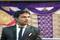Join the social network of Tech Nerds, increase skill rank, get work, manage projects...

• # Python TypeError: object of type 'NoneType' has no len()@rajjuja• 0
• 0
• 0
• 3
• 0
• 0
• 0
• 5.52k

I was unable to find what is wrong in the following code, The error msg is "object of type 'NoneType' has no len()"

My Code ------>

``````A=[31,22,14,15]

def merge(list1,list2):
output=[]
i=0
j=0
length1=len(list1)
length2=len(list2)

while i<length1 or j<length2:

if i<length1 and  j<length2:
if list1[i] < list2[j]:
output +=[list1[i]]
i +=1
else:
output +=[list2[j]]
j +=1

elif i<length1:
output +=[list1[i]]
i +=1

else:
output +=[list2[j]]
j+=1

def merge_sort(list1):
if len(list1)<=1:
return list1
else:
mid=int(len(list1)/2)
left =list1[:mid]
right=list1[mid:]
sorted_left=merge_sort(left)
sorted_right=merge_sort(right)
return merge(sorted_left,sorted_right)

def print_it(lista):
merge_sort(lista)
print "OutPut : ",output

print_it(A)``````

•@siva.singhcan you send me the file so i can run it my machine then i will check and make it correct and send it to you .
•@daniel.baboiuThe error is that merge() does not return anything (returns implicitly None), but its return value is returned by merge_sort(). Hence, checking the length of a None.

Also, print_it() uses print output, but output is not initialized in the scope of the function.
•@siva.singhHi, the code you were using is correct, only the issue is of "indentation error" which comes because of IDE you were using, I am using pycharm IDE. You can use this code:
``````A=[31,22,14,15]
def merge(list1,list2):
output=[]
i=0
j=0
length1=len(list1)
length2=len(list2)
while i<length1 or j<length2:

if i<length1 and  j<length2:
if list1[i] < list2[j]:
output +=[list1[i]]
i +=1
else:
output +=[list2[j]]
j +=1

elif i<length1:
output +=[list1[i]]
i +=1

else:
output +=[list2[j]]
j+=1

def merge_sort(list1):
if len(list1)<=1:
return list1
else:
mid=int(len(list1)/2)
left =list1[:mid]
right=list1[mid:]
sorted_left=merge_sort(left)
sorted_right=merge_sort(right)
return merge(sorted_left,sorted_right)
def print_it(lista):
merge_sort(lista)
print "OutPut : ",output

print_it(A)``````
OR
OR
Register

OR
Fill out the form below and instructions to reset your password will be emailed to you:

• Hire
• Post Projects

### Post Projects

• All at 0 Cost ....
• Post Tech Job
• Select Best Bidder
• Track the Project
• Approve Work and Pay safely
• Browse Nerds
• Work
• Find Projects Find Projects
• UI Design and UX
• Software Engineering
View more...
View less...
• Marketing
• General
• Manage
• Company Company

### Manage Company

• All at 0 Cost ....
• Manage Company and Employee Profiles
• Company wide Employee Productivity Reports
• Knowledge Sharing and Collaboration Tools
• Get Sales Lead and Bid for Tech Projects
• Send Invoices and Receive Payment Safely
• Learn
• Nerd Digest Nerd Digest
• UI Design and UX
• Software Engineering
View more...
View less...
• Marketing
• General
• Tech Q & A Tech Q & A
• UI Design and UX
• Software Engineering
View more...
View less...
• Marketing
• General